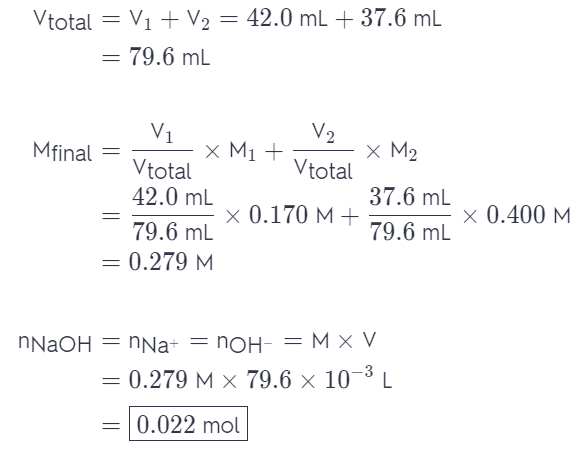# Indicate The Concentration Of Each Ion Present In The Solution Formed By Mixing

We thoroughly check each answer to a question to provide you with the most correct answers. Found a mistake? Let us know about it through the REPORT button at the bottom of the page.

Indicate the concentration of each ion present in the solution formed by mixing 42.0 mL of 0.170 M NaOH with 37.6 mL of 0.400 M NaOH,

Answer: nNaOH = nNa+ = nOH- = 0.022mol.

## Explanation

We can do this by calculating the volume and molarity of the final solution. Once we have that we can easily determine the amount of moles of each of the ions. With NaOH, the amount of NaOH will be the same as the amount of Na+ and OH- (ratio 1:1). Therefore: